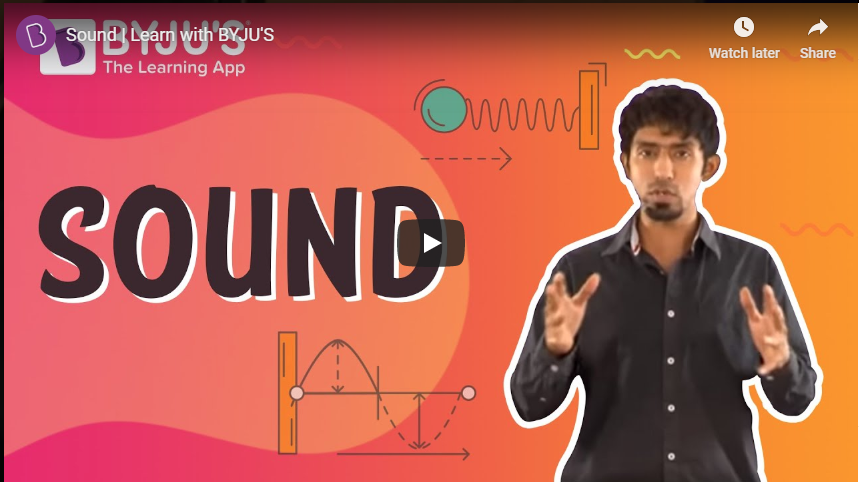# Scientific InvestigationAn investigation is the heart of Science. It is how scientists do research. Investigations provide an answer to questions and help us resolve problems. Even if the evidence doesn’t provide solutions, it may still be useful, leading to new issues for investigation. Science strides, as more knowledge is gathered. In this article, we will walk you through the scientific investigation, a logical problem-solving approach used by scientists.

## How to Make a Scientific Investigation?

At the heart of all sciences, there lies a problem-solving approach known as the scientific method. The scientific method has basic procedures as follows:

• Make an observation
• Form hypothesis
• Make predictions based on the hypothesis
• Test the prediction
• Iterate: use a new hypothesis or predictions

Scientists in different fields of Sciences ask different questions and perform different tests. However, the same core approach is used by scientists to find answers that are logical and supported by evidence.

### Scientific Investigation Example

Let us better understand scientific investigation by applying its steps to solve everyday problems.

1. Make an observation

Suppose you switch on your television at home to watch your favorite movie. However, your television doesn’t turn on.

Why didn’t my television switch on?

3. Propose a hypothesis

A potential answer to a question that can be tested is known as a hypothesis. The hypothesis, in this case, can be that television didn’t work because of the broken electrical outlet.

4. Make predictions

A prediction is an outcome we would expect to see if the hypothesis is correct. In this case, we would predict that if the electrical outlet is broken, then plugging the television into a different outlet should solve the problem.

5. Test the predictions

To test the hypothesis, we need to perform an experiment associated with the forecast. For instance, in this case, we would plug the television into a different outlet and see if it works.

• If the television switches on, then the hypothesis is likely to be correct.
• If the television doesn’t switch on, then the hypothesis is likely to be wrong.

Unless there was a flaw in the test, a contradicting result implies that we should reject the hypothesis and look for a new one.

6. Iterate

The last step is to reflect on our results and use them to guide our next steps.

• If the hypothesis was correct, then we might have to perform a further test to confirm it to be more explicit. For example, why did the outlet break?
• If it were not supported, then we would have to develop a new hypothesis. For example, there is a broken circuit on television.

In most circumstances, scientific investigation is an iterative process. It is a cycle rather than a straight line.